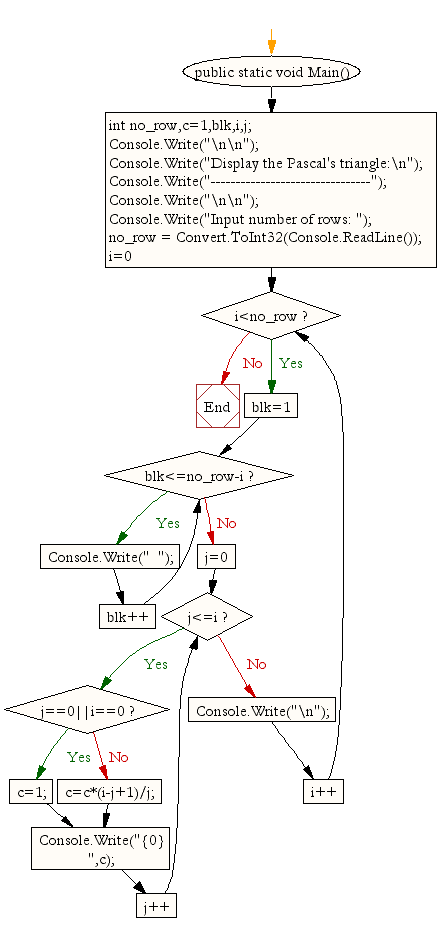﻿ C# - Display by Pascal's triangle - w3resource# C# Sharp Exercises: Display by Pascal's triangle

## C# Sharp For Loop: Exercise-33 with Solution

Write a C# Sharp Program to display by Pascal's triangleSample Solution:-

C# Sharp Code:

``````using System;
public class Exercise33
{
public static void Main()
{
int no_row,c=1,blk,i,j;

Console.Write("\n\n");
Console.Write("Display the Pascal's triangle:\n");
Console.Write("--------------------------------");
Console.Write("\n\n");

Console.Write("Input number of rows: ");
for(i=0;i<no_row;i++)
{
for(blk=1;blk<=no_row-i;blk++)
Console.Write("  ");
for(j=0;j<=i;j++)
{
if (j==0||i==0)
c=1;
else
c=c*(i-j+1)/j;
Console.Write("{0}   ",c);
}
Console.Write("\n");
}
}
}
```
```

Sample Output:

```Display the Pascal's triangle:
--------------------------------
Input number of rows: 8
1
1   1
1   2   1
1   3   3   1
1   4   6   4   1
1   5   10   10   5   1
1   6   15   20   15   6   1
1   7   21   35   35   21   7   1
```

Flowchart:C# Sharp Code Editor:

Contribute your code and comments through Disqus.

What is the difficulty level of this exercise?

Test your Programming skills with w3resource's quiz.

﻿